LW = 'linewidth'; lw = 1.6;
MS = 'markersize'; ms = 12;
FS = 'fontsize'; fs = 12;

Here is the characteristic function on the interval $[-1/2,1/2]$:

x = chebfun('x',[-0.5 0.5]);
B0 = 1+0*x;
ax = [-3 3 -.2 1.2];
hold off, plot(B0,LW,lw), axis(ax), grid on
pts = [-.5 .5];
hold on, plot(pts,B0(pts,'left'),'.k',MS,ms)
plot(pts,B0(pts,'right'),'.k',MS,ms)
title('B-spline of order 0',FS,fs)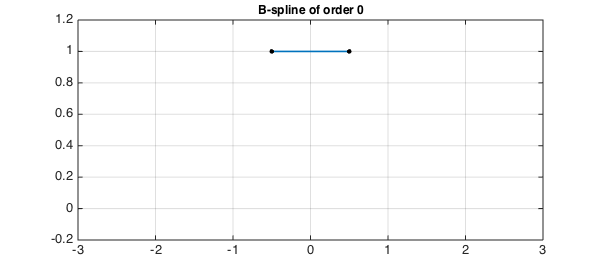If we convolve B0 with itself, we get a hat function:

B1 = conv(B0,B0);
hold off, plot(B1,LW,lw), axis(ax), grid on
pts = [pts-.5 max(pts)+.5];
hold on, plot(pts,B1(pts),'.k',MS,ms)
title('B-spline of order 1',FS,fs)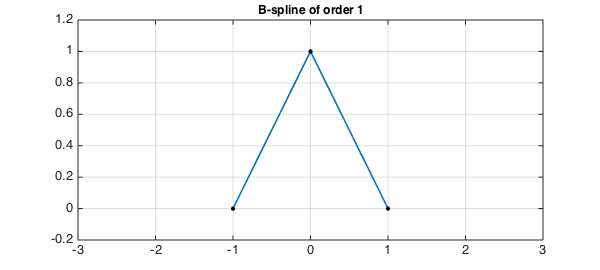Convolving this result with B0 gives us a $C^1$ function, piecewise parabolic:

B2 = conv(B0,B1);
hold off, plot(B2,LW,lw), axis(ax), grid on
pts = [pts-.5 max(pts)+.5];
hold on, plot(pts,B2(pts),'.k',MS,ms)
title('B-spline of order 2',FS,fs)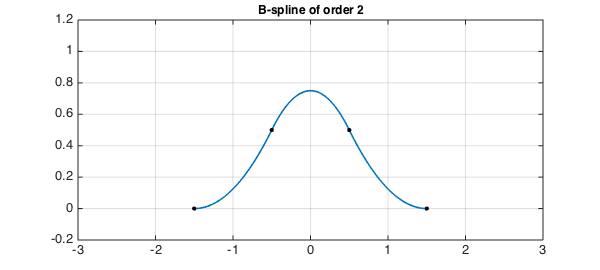As the titles of the plots indicate, these functions are known as B-splines. In our notation the B-spline $B_n$ is a $C^{n-1}$ piecewise polynomial of degree $n$ with support $[-(n+1)/2,(n+1)/2]$ and breakpoints uniformly spaced with separation $1$ this interval. The B-splines form a good basis for numerical computation with splines. Here is B3:

B3 = conv(B0,B2);
hold off, plot(B3,LW,lw), axis(ax), grid on
pts = [pts-.5 max(pts)+.5];
hold on, plot(pts,B3(pts),'.k',MS,ms)
title('B-spline of order 3',FS,fs)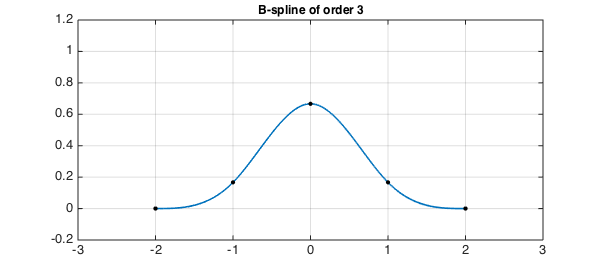And here is B4:

B4 = conv(B0,B3);
hold off, plot(B4,LW,lw), axis(ax), grid on
pts = [pts-.5 max(pts)+.5];
hold on, plot(pts,B4(pts),'.k',MS,ms)
title('B-spline of order 4',FS,fs)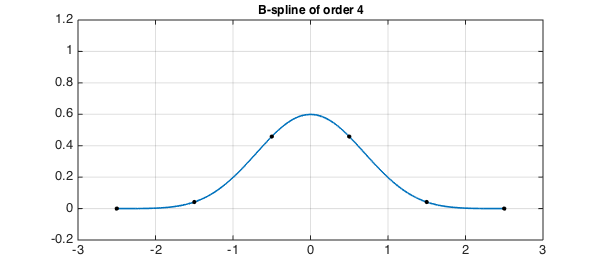B-splines were introduced by Schoenberg and became a standard method for numerical computation following the work of de Boor  and Cox  in 1972. In general there is no need for the breakpoints to be uniformly spaced. With uniformly spaced points as in our example, however, the shape approaches a Gaussian as $n\to\infty$. One could derive this fact by noting that in Fourier space, each convolution is a multiplication by the Fourier transform of $B_0$, namely a sinc function. This is the same computation that arises in the proof of the Central Limit Theorem, and in fact, the B-splines have a probabilistic interpretation: if $B_0$ represents a random variable with uniform distribution on the interval $[-1/2,1/2]$ then $B_1$ is the distribution for the sum of two independent samples from $B_0$, $B_2$ is the distribution for the sum of three samples from $B_0$, and so on.

1. C. de Boor, On calculating with B-splines, Journal of Approximation Theory, 6 (1972), 50-62.

2. M. Cox, The numerical evaluation of B-splines, Journal of the Institute of Mathematics and its Applications, 10 (1972), 134-149.